# Linear Programming and Parameterized Algorithms Linear Programming n

• Slides: 55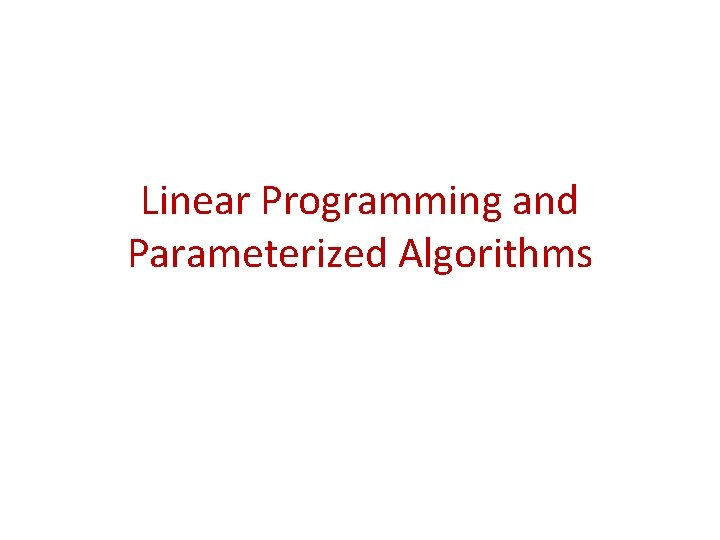Linear Programming and Parameterized AlgorithmsLinear Programming n real-valued variables, x 1, x 2, … , xn. Linear objective function. Linear (in)equality constraints. Solvable in polynomial time.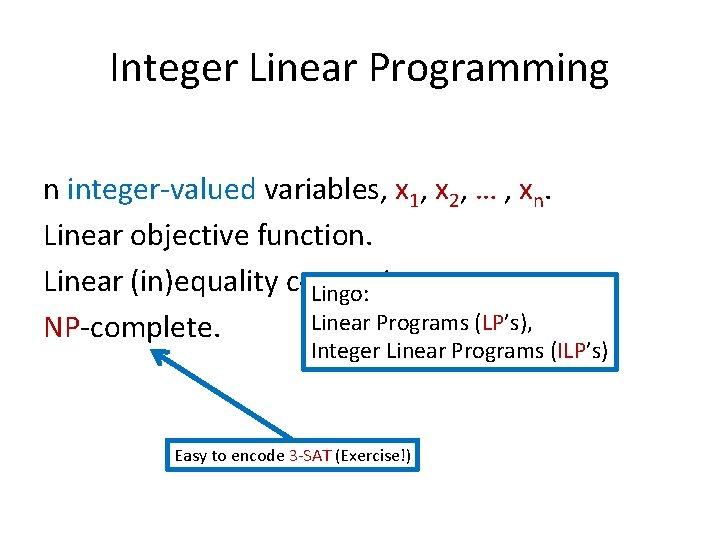Integer Linear Programming n integer-valued variables, x 1, x 2, … , xn. Linear objective function. Linear (in)equality constraints. Lingo: Linear Programs (LP’s), NP-complete. Integer Linear Programs (ILP’s) Easy to encode 3 -SAT (Exercise!)Vertex Cover Have seen a kernel with O(k 2) vertices, will see a kernel with 2 k vertices.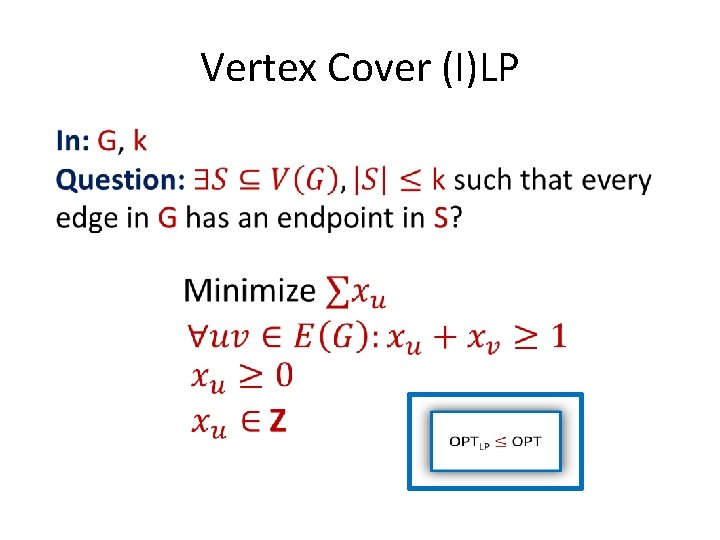Vertex Cover (I)LP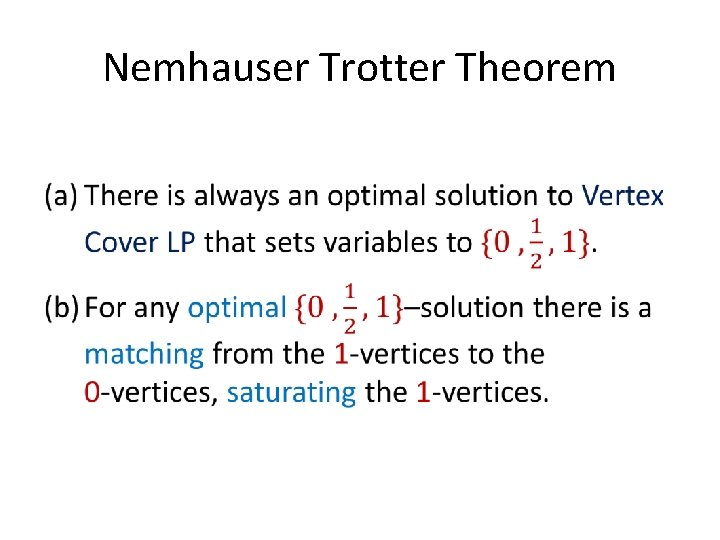Nemhauser Trotter Theorem •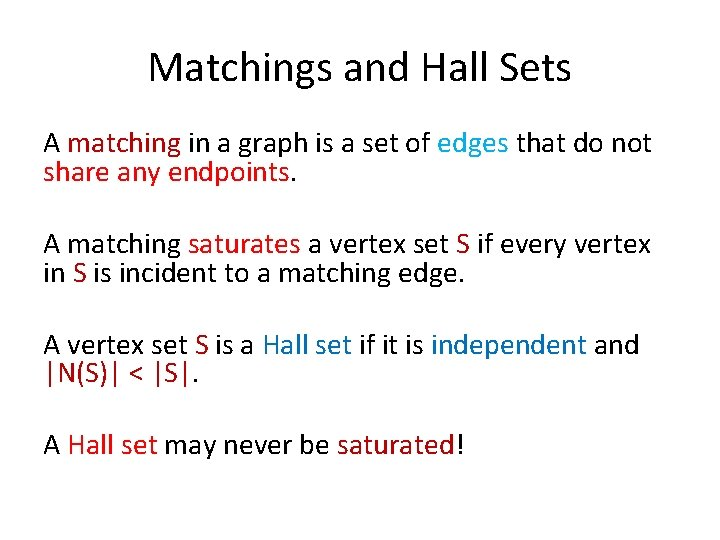Matchings and Hall Sets A matching in a graph is a set of edges that do not share any endpoints. A matching saturates a vertex set S if every vertex in S is incident to a matching edge. A vertex set S is a Hall set if it is independent and |N(S)| < |S|. A Hall set may never be saturated!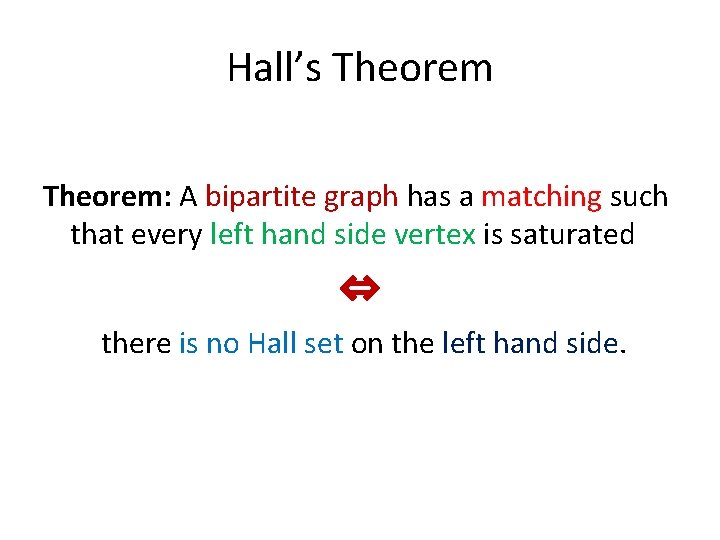Hall’s Theorem: A bipartite graph has a matching such that every left hand side vertex is saturated ⇔ there is no Hall set on the left hand side.Hall’s Theorem Example Matching (so no Hall set) Hall set (so no matching)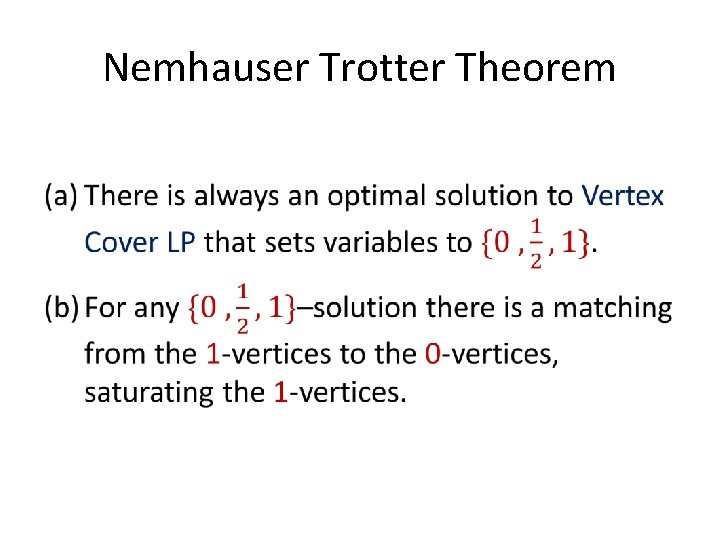Nemhauser Trotter Theorem •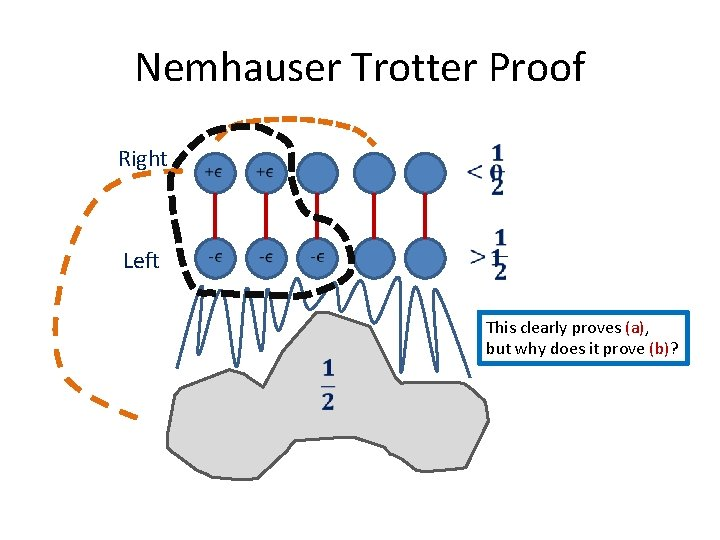Nemhauser Trotter Proof Right Left This clearly proves (a), but why does it prove (b)?Reduction Rule If exists optimal LP solution that sets xv to 1, then exists optimal vertex cover that selects v. Remove v from G and decrease k by 1. Correctness follows from Nemhauser Trotter Polynomial time by LP solving.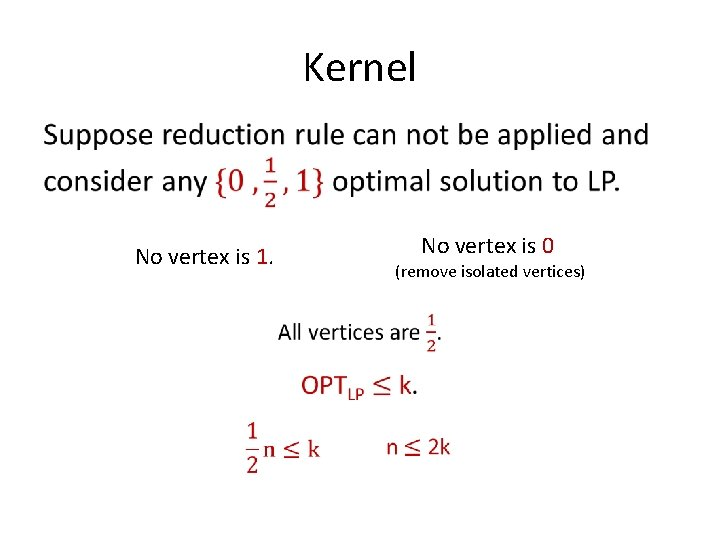Kernel • No vertex is 0 No vertex is 1. (remove isolated vertices)Above LP Vertex Cover •Vertex Cover Above LP •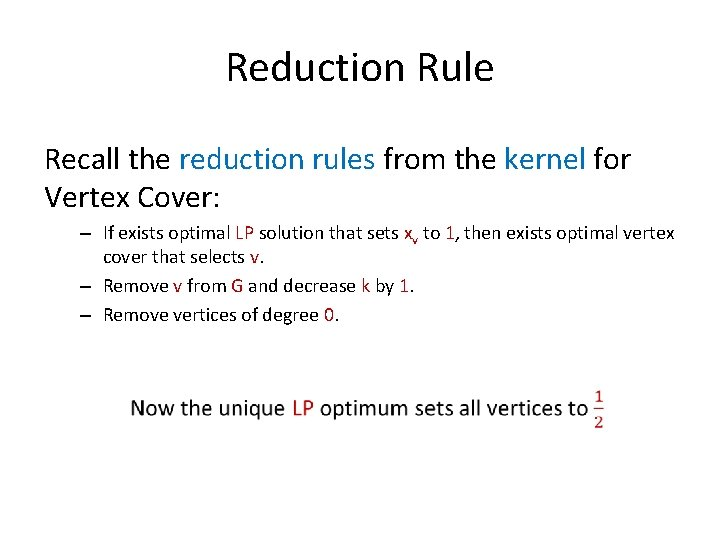Reduction Rule Recall the reduction rules from the kernel for Vertex Cover: – If exists optimal LP solution that sets xv to 1, then exists optimal vertex cover that selects v. – Remove v from G and decrease k by 1. – Remove vertices of degree 0.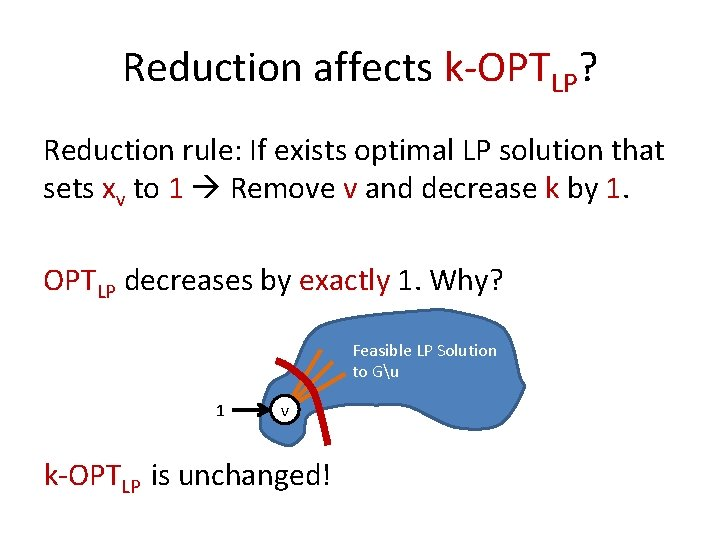Reduction affects k-OPTLP? Reduction rule: If exists optimal LP solution that sets xv to 1 Remove v and decrease k by 1. OPTLP decreases by exactly 1. Why? Feasible LP Solution to Gu 1 v k-OPTLP is unchanged!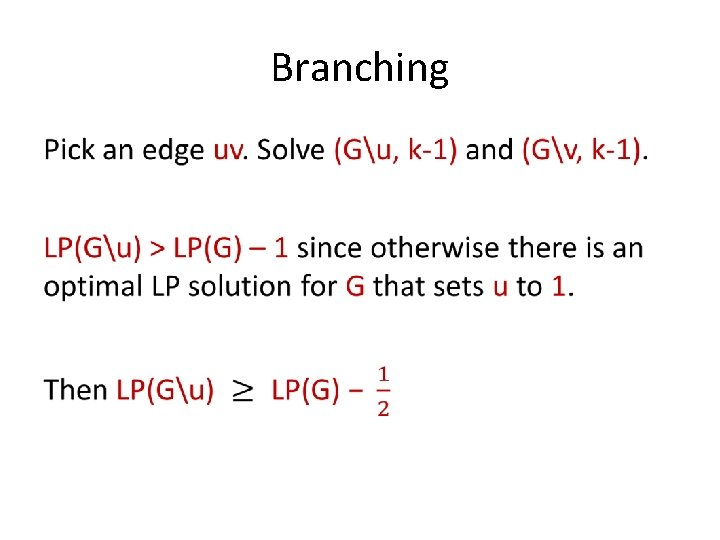Branching •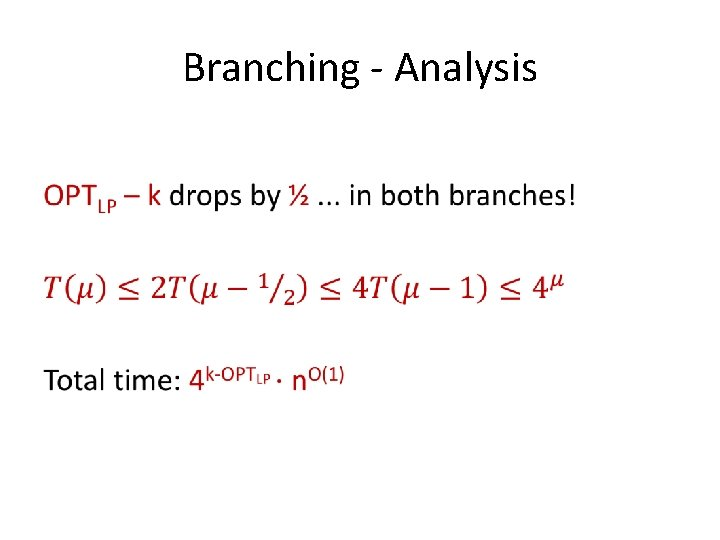Branching - Analysis •Vertex Cover recap • Is this useful when compared to a 1. 38 k algorithm?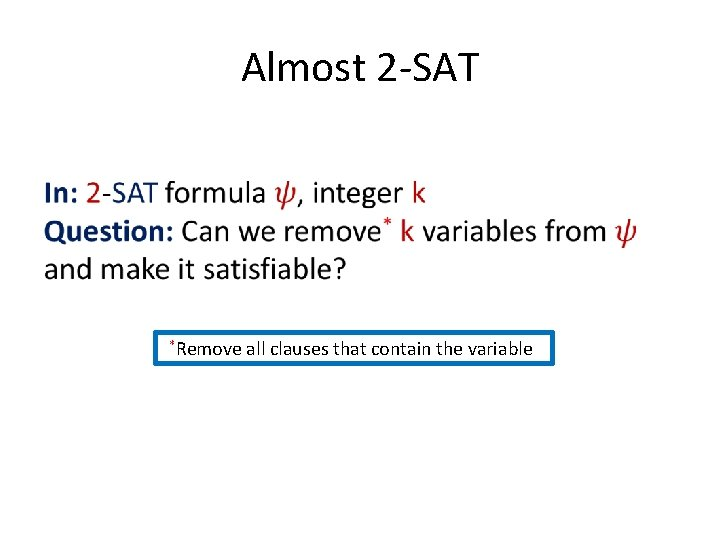Almost 2 -SAT *Remove all clauses that contain the variableOdd Cycle Transversal (OCT) Will give algorithms for Almost 2 -SAT and OCT, using FPT-reductions to Vertex Cover above LP!Odd Cycle Transversal Almost 2 -Sat x y z z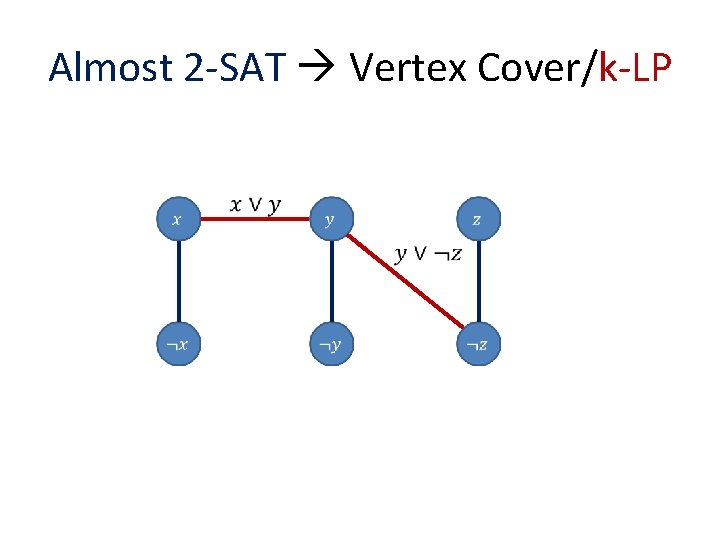Almost 2 -SAT Vertex Cover/k-LP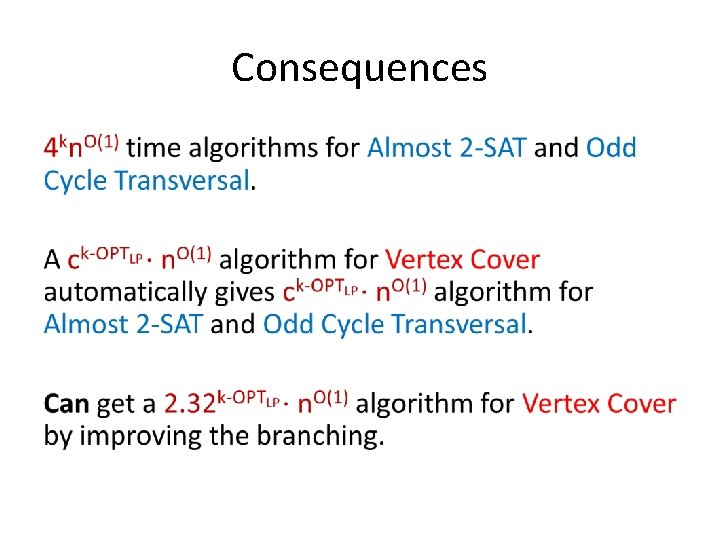Consequences •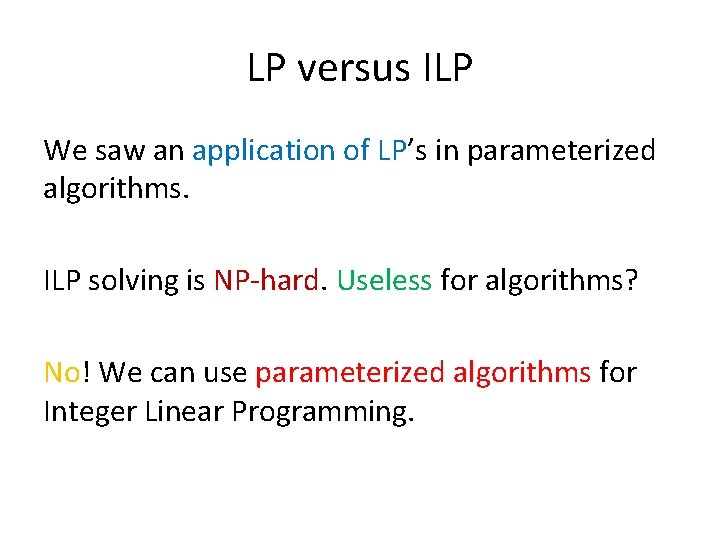LP versus ILP We saw an application of LP’s in parameterized algorithms. ILP solving is NP-hard. Useless for algorithms? No! We can use parameterized algorithms for Integer Linear Programming.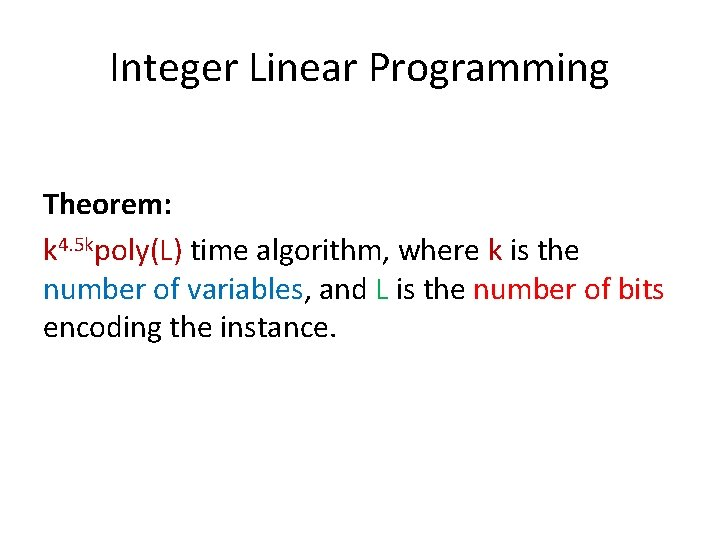Integer Linear Programming Theorem: k 4. 5 kpoly(L) time algorithm, where k is the number of variables, and L is the number of bits encoding the instance.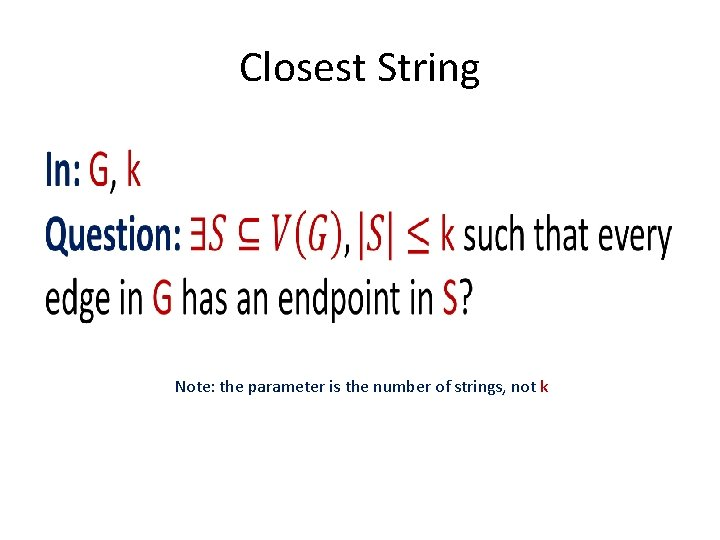Closest String • Note: the parameter is the number of strings, not k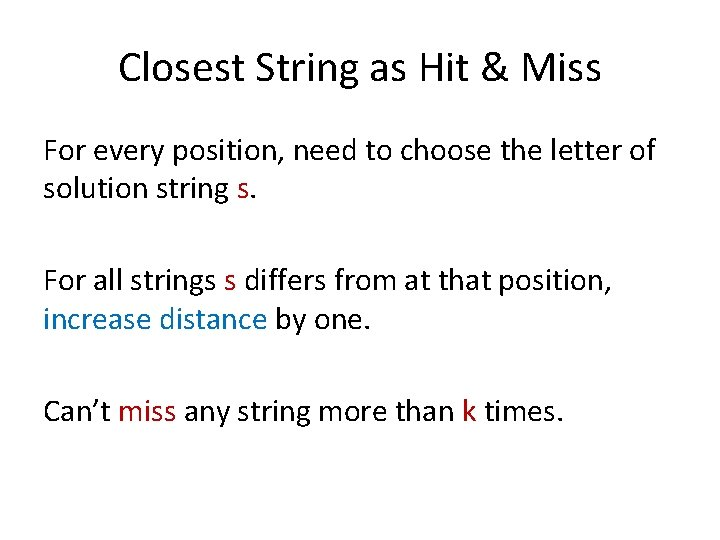Closest String as Hit & Miss For every position, need to choose the letter of solution string s. For all strings s differs from at that position, increase distance by one. Can’t miss any string more than k times.Closest String Alphabet Reduction Can assume that alphabet size is at most n. 11111111 123412342222 321443322114 11111111 122212222222 221223232113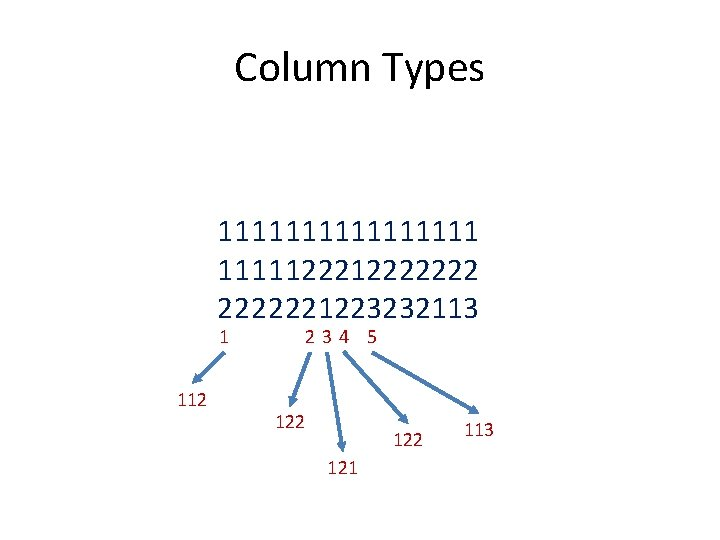Column Types 1111111122222221223232113 1 112 234 5 122 121 113Closest String ILP After alphabet reduction, there at most nn column types. Count the number of columns of each column type.ILP For each column type, make n variables, one for each letter. Constraints: For each column type t, the chosen letters add up to the number of type t.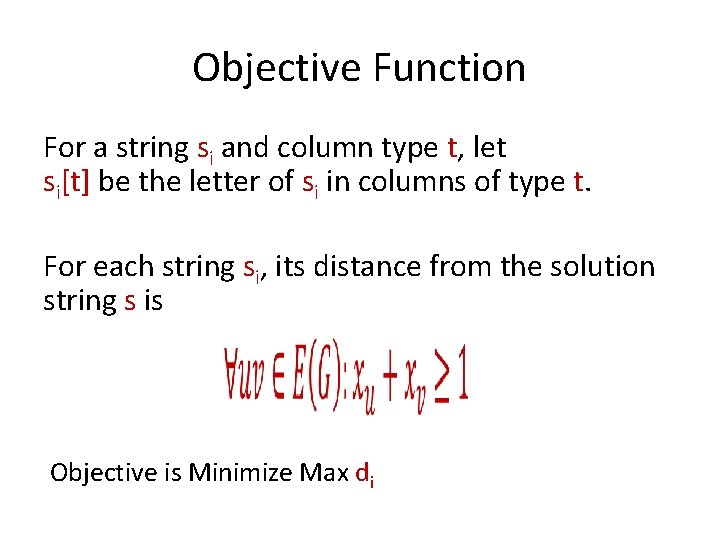Objective Function For a string si and column type t, let si[t] be the letter of si in columns of type t. For each string si, its distance from the solution string s is Objective is Minimize Max di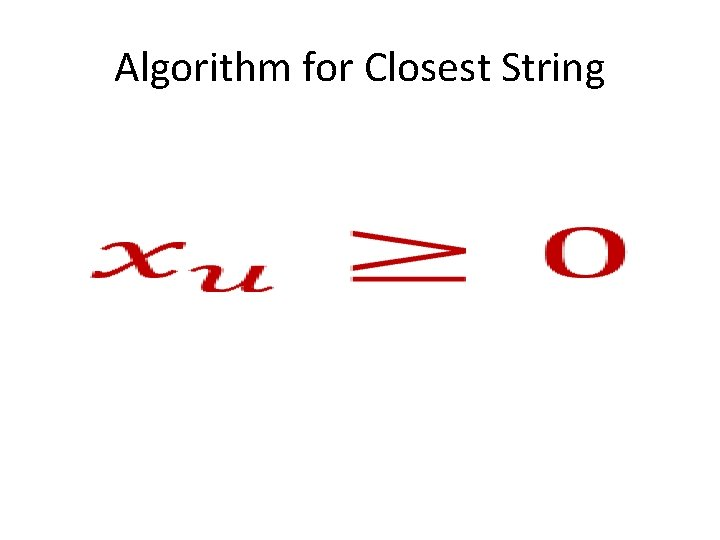Algorithm for Closest String •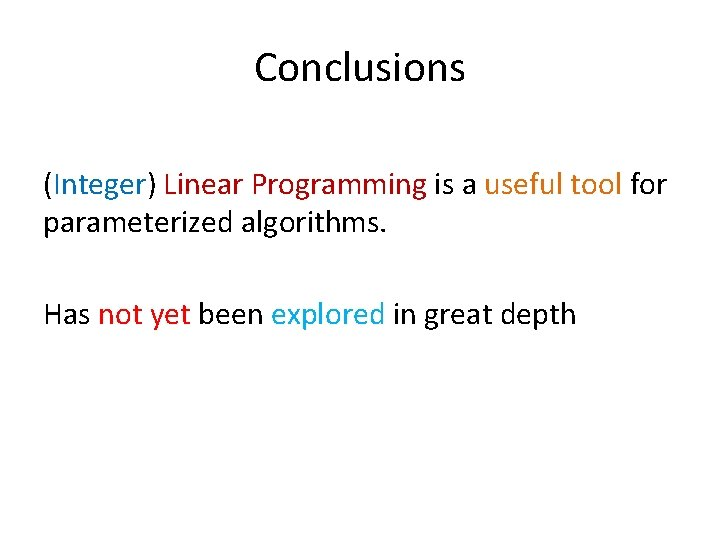Conclusions (Integer) Linear Programming is a useful tool for parameterized algorithms. Has not yet been explored in great depth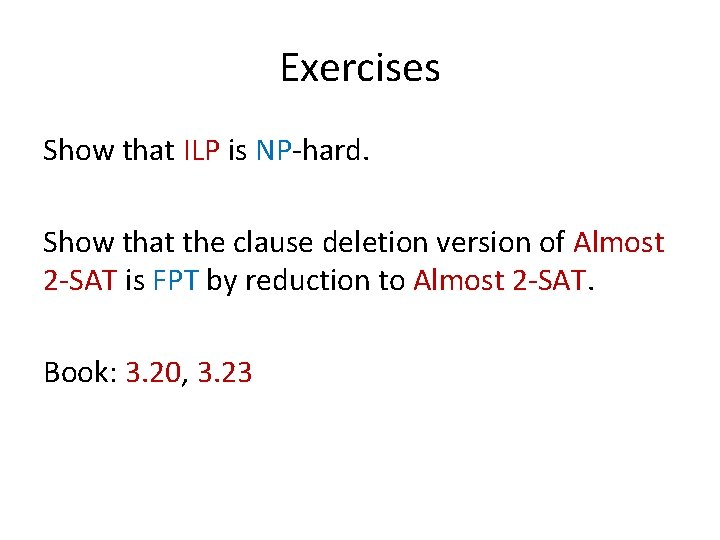Exercises Show that ILP is NP-hard. Show that the clause deletion version of Almost 2 -SAT is FPT by reduction to Almost 2 -SAT. Book: 3. 20, 3. 23Thank You?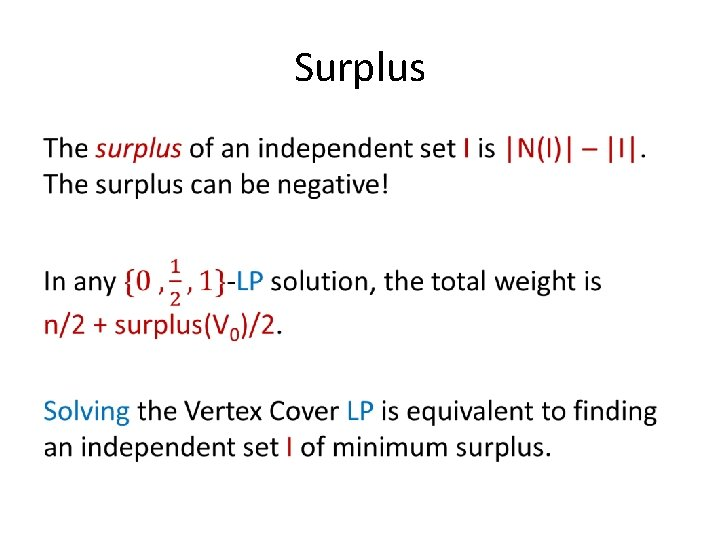Surplus •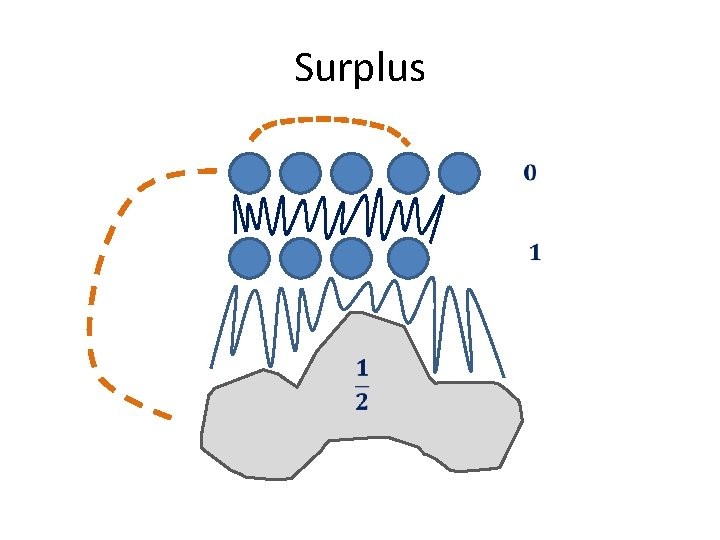SurplusSurplus and Reductions If «all ½» is the unique LP optimum then surplus(I) > 0 for all independent sets. Can we say anything meaningful for independent sets of surplus 1? 2? k?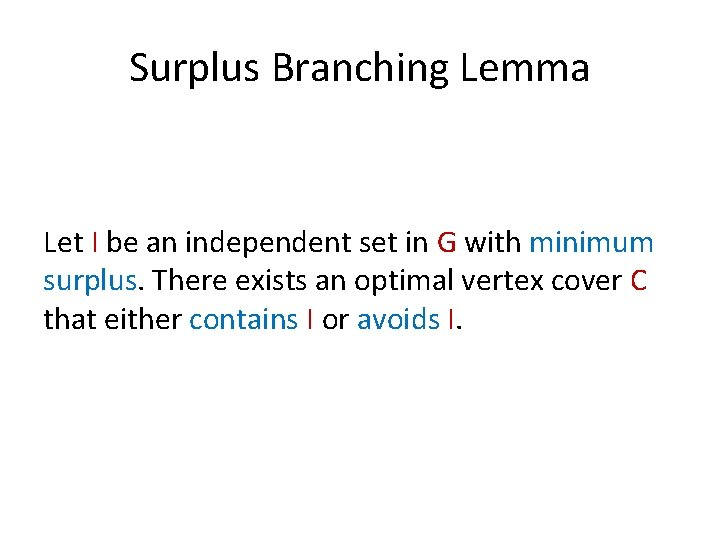Surplus Branching Lemma Let I be an independent set in G with minimum surplus. There exists an optimal vertex cover C that either contains I or avoids I.Surplus Branching Lemma Proof I N(I) RBranching Rule Find an independent set I of minimum surplus. Branch: Either put all of I into solution, or put all of N(I) into solution. Correct by branching lemma. Need to analyze measure drop in both branches.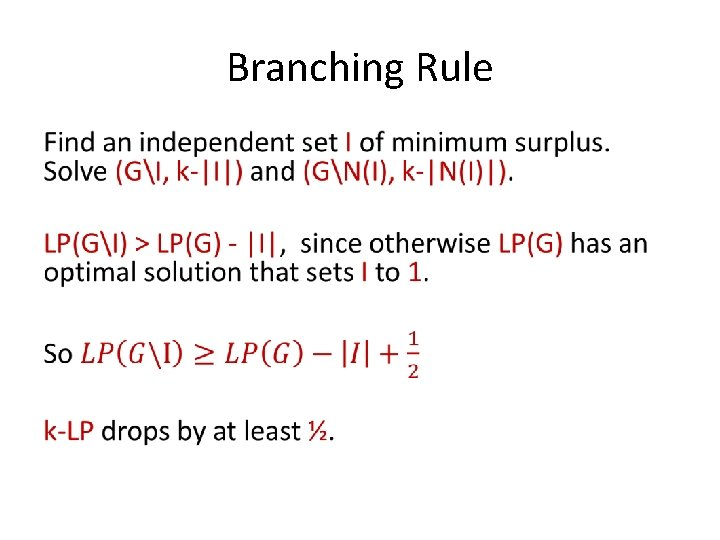Branching Rule •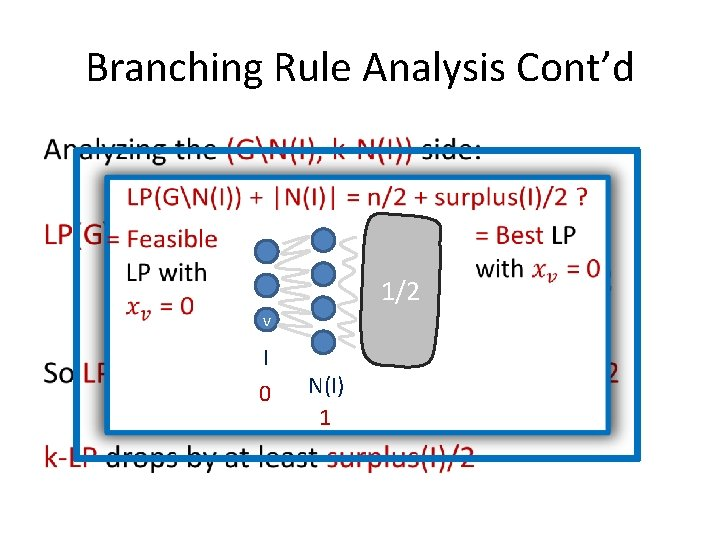Branching Rule Analysis Cont’d • 1/2 v I 0 N(I) 1Branching Summary •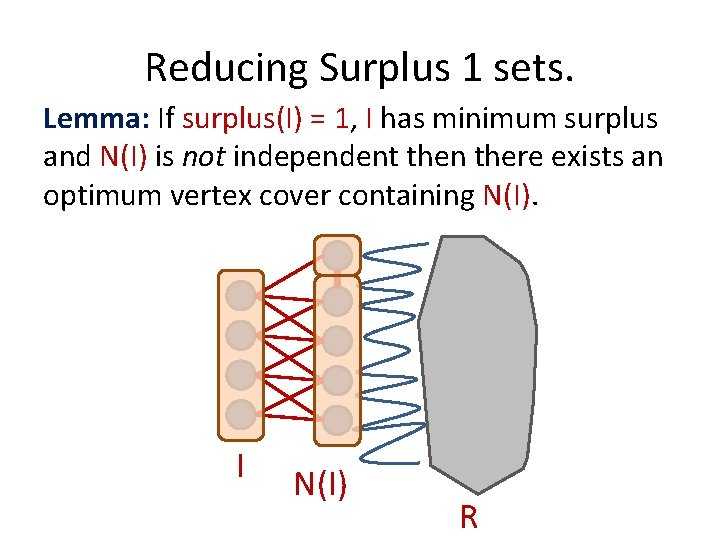Reducing Surplus 1 sets. Lemma: If surplus(I) = 1, I has minimum surplus and N(I) is not independent then there exists an optimum vertex cover containing N(I). I N(I) R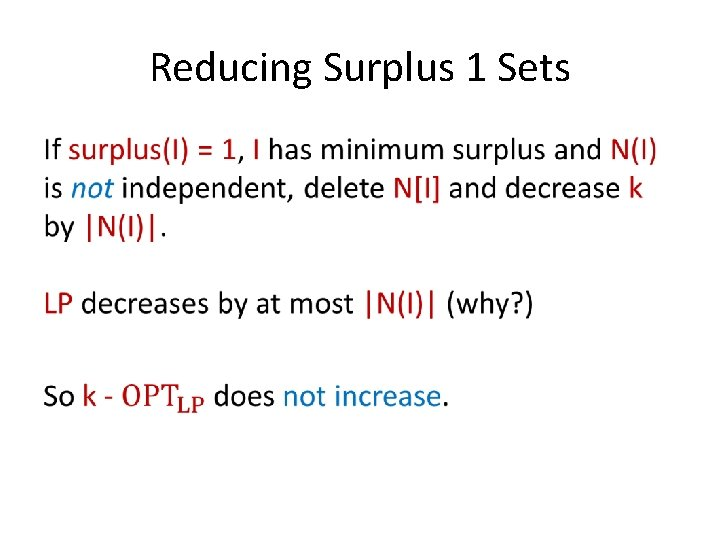Reducing Surplus 1 Sets •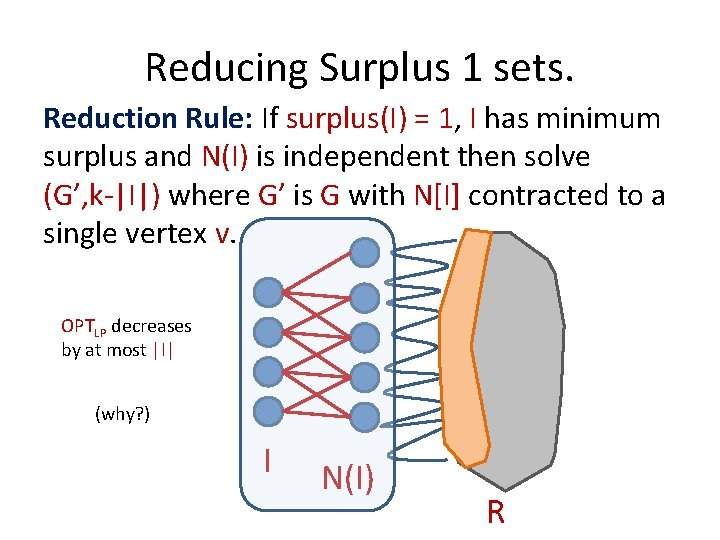Reducing Surplus 1 sets. Reduction Rule: If surplus(I) = 1, I has minimum surplus and N(I) is independent then solve (G’, k-|I|) where G’ is G with N[I] contracted to a single vertex v. OPTLP decreases by at most |I| (why? ) I N(I) RSummary • The correctness of these rules were also proved by NT!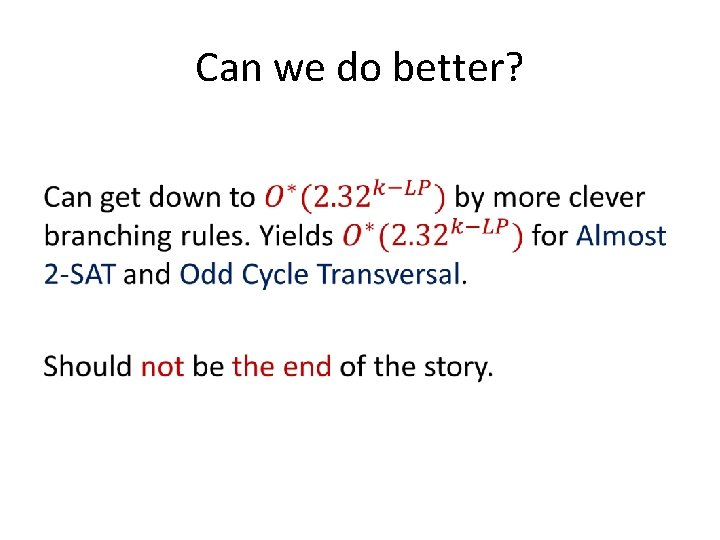Can we do better? •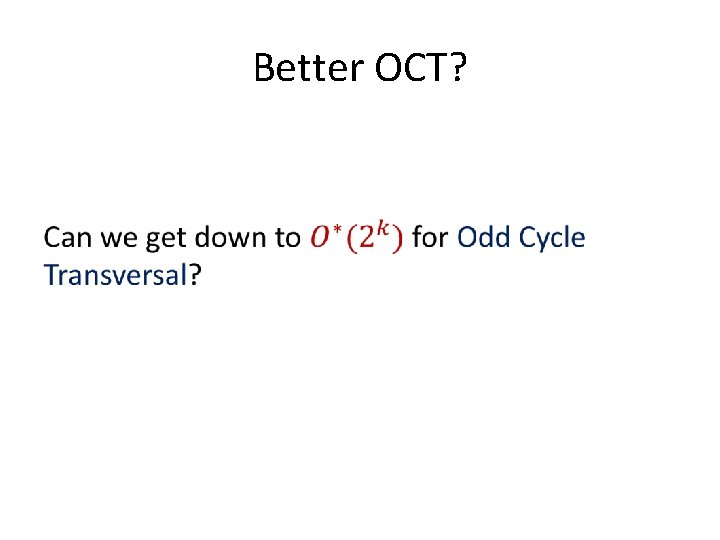Better OCT? •LP Branching in other cases I believe more problems should have FPT algorithms by LP-guided branching. One example: Multiway Cut. What about. . . (Directed) Feedback Vertex Set, parameterized by solution size k?Thank You!